Anzeige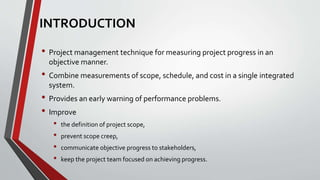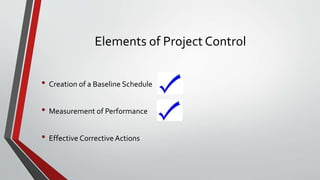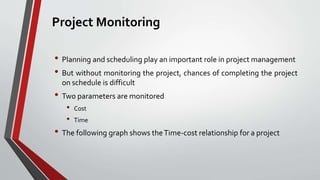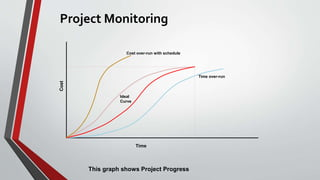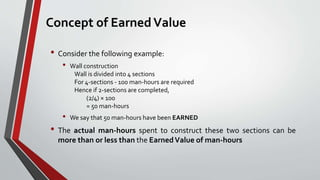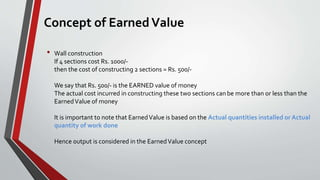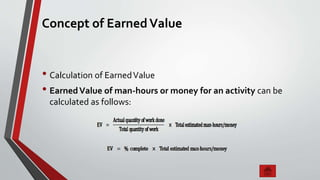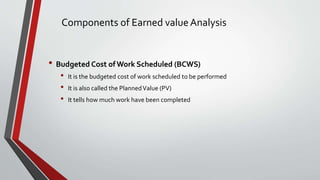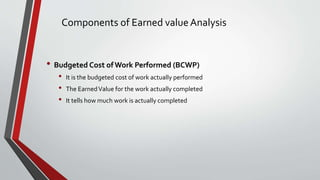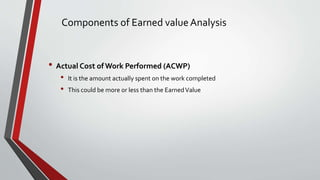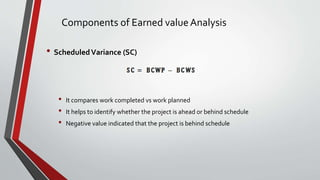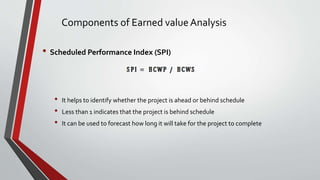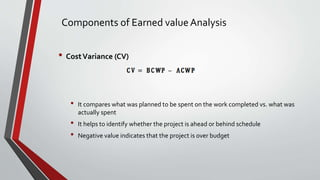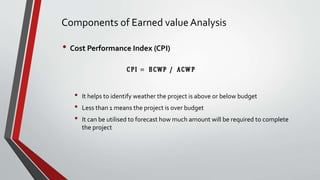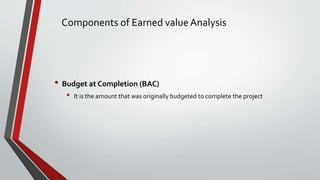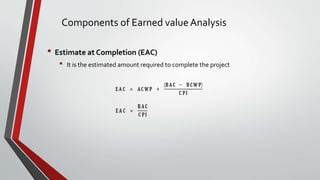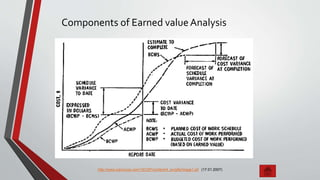1 von 22
Anzeige

### Earned value management

1. EARNED VALUE MANAGEMENT ASHIF A R ashifmsd@gmail.com Ph No. - 9745350127
2. INTRODUCTION • Project management technique for measuring project progress in an objective manner. • Combine measurements of scope, schedule, and cost in a single integrated system. • Provides an early warning of performance problems. • Improve • the definition of project scope, • prevent scope creep, • communicate objective progress to stakeholders, • keep the project team focused on achieving progress.
3. Elements of Project Control • Creation of a Baseline Schedule • Measurement of Performance • Effective Corrective Actions
4. Project Monitoring
5. Project Monitoring • Planning and scheduling play an important role in project management • But without monitoring the project, chances of completing the project on schedule is difficult • Two parameters are monitored • Cost • Time • The following graph shows the Time-cost relationship for a project
6. Project Monitoring Cost Cost over-run with schedule Time Time over-run Ideal Curve This graph shows Project Progress
7. Concepts of Earned Value
8. Concept of Earned Value • Consider the following example: • Wall construction Wall is divided into 4 sections For 4-sections - 100 man-hours are required Hence if 2-sections are completed, (2/4) × 100 = 50 man-hours • We say that 50 man-hours have been EARNED • The actual man-hours spent to construct these two sections can be more than or less than the EarnedValue of man-hours
9. Concept of Earned Value • Wall construction If 4 sections cost Rs. 1000/- then the cost of constructing 2 sections = Rs. 500/- We say that Rs. 500/- is the EARNED value of money The actual cost incurred in constructing these two sections can be more than or less than the Earned Value of money It is important to note that Earned Value is based on the Actual quantities installed or Actual quantity of work done Hence output is considered in the Earned Value concept
10. Concept of Earned Value • Calculation of Earned Value • Earned Value of man-hours or money for an activity can be calculated as follows:
11. Components of Earned Value
12. Components of Earned value Analysis • Budgeted Cost ofWork Scheduled (BCWS) • It is the budgeted cost of work scheduled to be performed • It is also called the Planned Value (PV) • It tells how much work have been completed
13. Components of Earned value Analysis • Budgeted Cost ofWork Performed (BCWP) • It is the budgeted cost of work actually performed • The Earned Value for the work actually completed • It tells how much work is actually completed
14. Components of Earned value Analysis • Actual Cost ofWork Performed (ACWP) • It is the amount actually spent on the work completed • This could be more or less than the Earned Value
15. Components of Earned value Analysis • Scheduled Variance (SC) • It compares work completed vs work planned • It helps to identify whether the project is ahead or behind schedule • Negative value indicated that the project is behind schedule
16. Components of Earned value Analysis • Scheduled Performance Index (SPI) • It helps to identify whether the project is ahead or behind schedule • Less than 1 indicates that the project is behind schedule • It can be used to forecast how long it will take for the project to complete
17. Components of Earned value Analysis • Cost Variance (CV) • It compares what was planned to be spent on the work completed vs. what was actually spent • It helps to identify whether the project is ahead or behind schedule • Negative value indicates that the project is over budget
18. Components of Earned value Analysis • Cost Performance Index (CPI) • It helps to identify weather the project is above or below budget • Less than 1 means the project is over budget • It can be utilised to forecast how much amount will be required to complete the project
19. Components of Earned value Analysis • Budget at Completion (BAC) • It is the amount that was originally budgeted to complete the project
20. Components of Earned value Analysis • Estimate at Completion (EAC) • It is the estimated amount required to complete the project
21. Components of Earned value Analysis http://www.iceincusa.com/16CSP/content/4_ev/gifs/image1.gif (17.01.2007)
Anzeige